# Featured Products

Newton’s Laws Revisited

Newton’s Principia Mathematica, the book published in 1687, contains Newton’s three laws of motion. Most people think he wrote it to explain forces on blocks, falling stones, and pulleys. But that’s not true. The purpose of the book was to explain the motion of the planets and comets. Ultimately, Newton proves that Kepler’s “laws” of planetary motion are actually a natural consequence of one single law of the inverse square relationship of gravitational force.

In the preface to the book, Newton distinguishes between Practical Mechanics, which would include fixing windmills and carts (today automobiles), and Rational Mechanics, which concerns itself with the motion of the planets and the Earth among the stars (Fig. 2). These days Rational Mechanics is called Celestial Mechanics. It was Newton’s breakthrough and his thesis was that the laws of physics would be the same whether on earth or up in the heavens. This is the apple story that the gravity on the apple would also apply to the moon. That is the gravity of the earth reaches beyond the earth’s surface, as far as you like into space, and pulls the moon into a curved path. In this video and article, I revisit Newton’s laws from within the historical context and from his own original purpose of devising them. To explain the motion of the planets. Frequently he speaks of “motion” as the product of mass and velocity, these days we would call this momentum.

1st Law

Although you already know it, I believe it is best if we restate it as follows:

All objects, including planets and meteors, will continue in their motion, in a straight line at constant speed, unless there is a force that acts on them.

On earth, we rarely see this event because there is so often a force, like friction, pushing or pulling on objects. Newton correctly hypothesized that there would be no air in outer space, and this would explain how the planets could continue in perpetual motion along their paths. He reasoned that there must be no air beyond the atmosphere near earth’s surface because as we climb mountains there is less and less air. At distances such as the moon (60 earth radii) there would be so little air that the moon’s motion would essentially be through a vacuum. This explains how it can move with no friction.

2nd Law

An alteration from linear motion is caused by a force (a push or a pull) but the larger the mass, the less the force will change the motion.

a = F / m

It is best to write the equation in this form, at least initially, to demonstrate the proportionalities. Alterations of motion are caused by force but mass (inertia) resists. As always, a force is a push or a pull. An object like Jupiter might be traveling through space and have its straight-line path altered by the inward pull of gravity caused by the sun.

Now it is no longer moving in a straight line. This relationship, a=F/m defines inertia as the effort required to alter an object’s motion, and it turns out that inertia can be measured by mass, which Newton called the quantity of matter.

In our physics classes, we often overemphasize acceleration as a solution to this formula. Then we get surprised when students don’t recognize changes in direction as alterations of motion encompassed by the definition of acceleration.

In a typical lab, somebody might let a Fan Cart go, and measure the acceleration difference as the mass is increased. But speeding up is only one form of alteration of motion. When I teach, I emphasize “alteration” not only because it is a more accurate word, but because it reflects Newton’s original meaning. Even the translator agrees with me (Figure 7).

Motion in a circle is a very good example of a motion that is being altered. An object that is not going in a straight line will necessarily be subject to a force. In the video, I show one of our devices, the “Exploring Newton’s First Law: Inertia Kit” which I use here to demonstrate that straight line at constant speed will happen if the force applied stops.

Newton’s second law can also explain why all objects fall at the same rate independent of mass, even a book and a piece of paper.   Normally, they do not succeed at falling at the same rate because of air friction on the paper. But a book and a piece of paper will fall at the same rate if we evacuated the room, but there is an easier way. If you put the paper on top of the book, then the book will push the air out of the way for them and they will fall together. A piece of paper will fall at the same rate as a book. We see the solution here.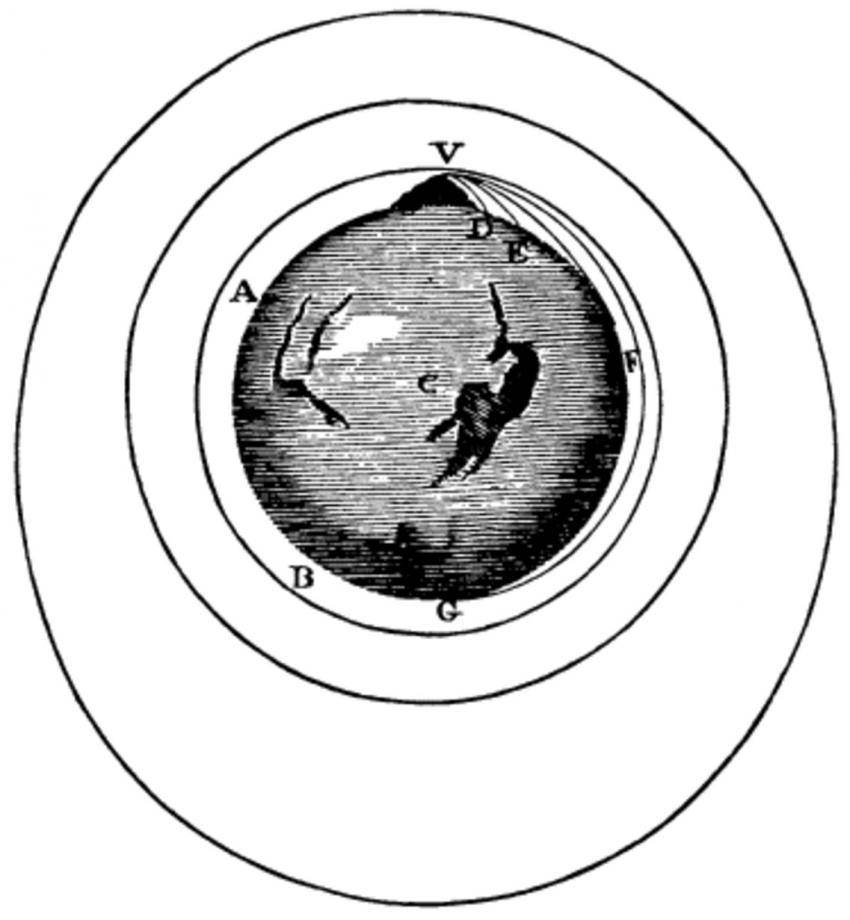Figure 1. Newton’s famous diagram which explains satellite orbits. Imagine a cannon is fired from a mountaintop. It could go fast enough to fall around the earth rather than upon it.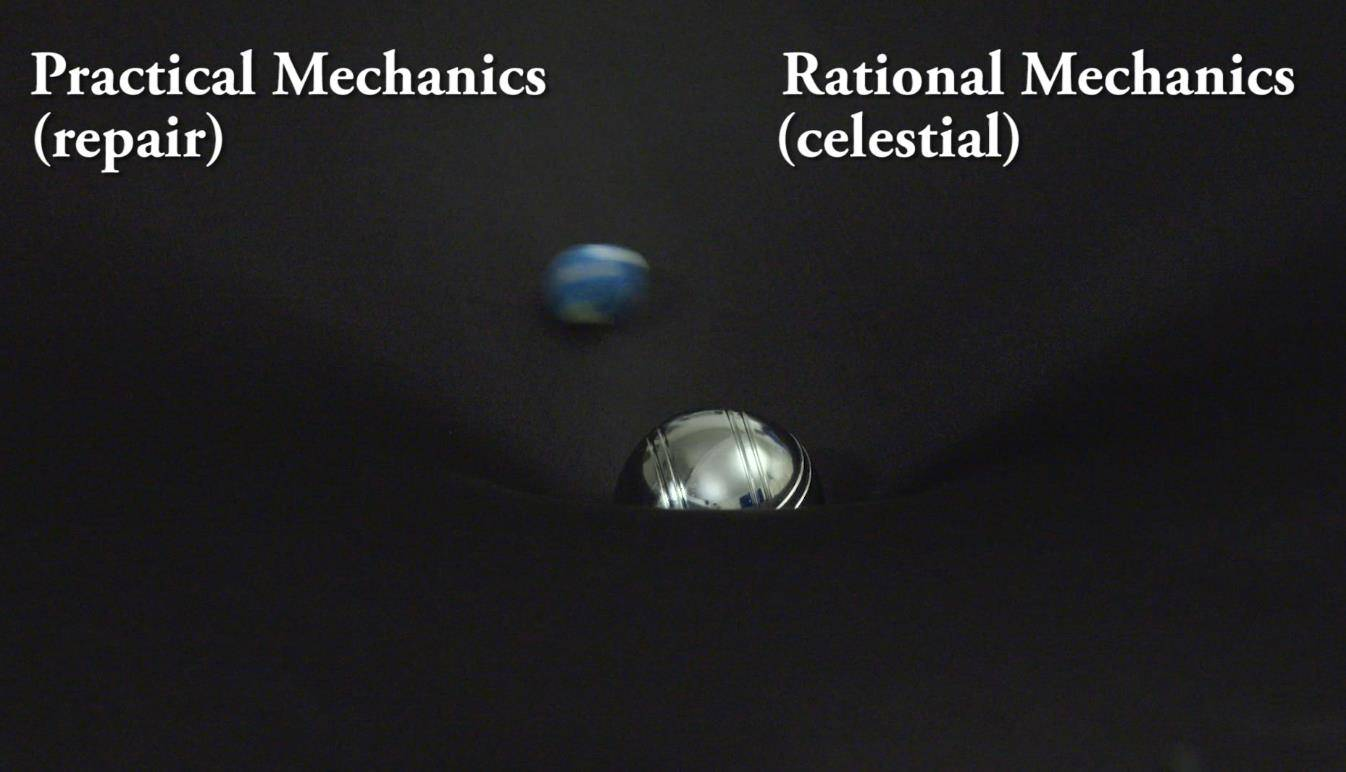Figure 2. Practical Mechanics and Rational Mechanics in one picture. Perhaps there is only one type of mechanics after all? A Gravity Well was used in this demonstration.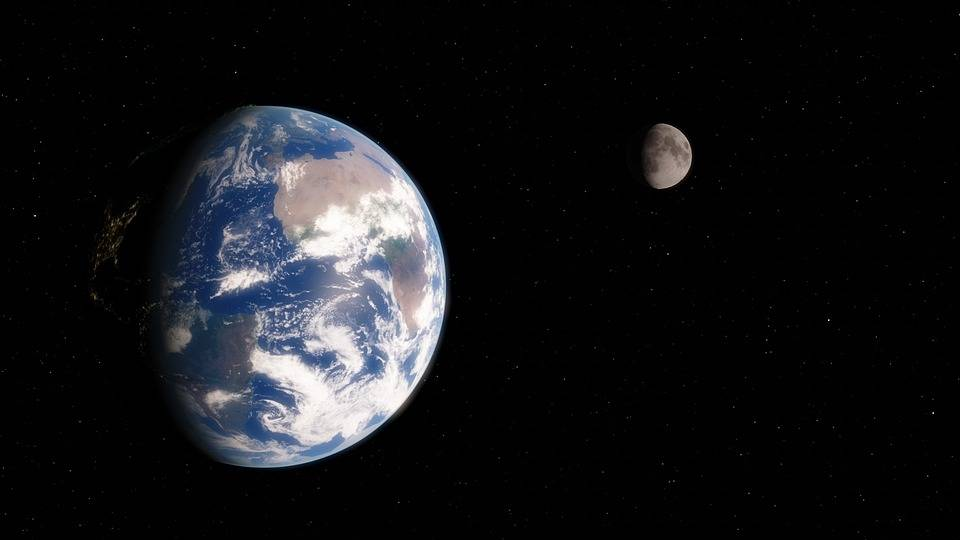Figure 3. Although Newton never had this view, we can see that the earth’s atmosphere barely extends beyond its surface. The moon should be much further off, about 10 feet away on this scale.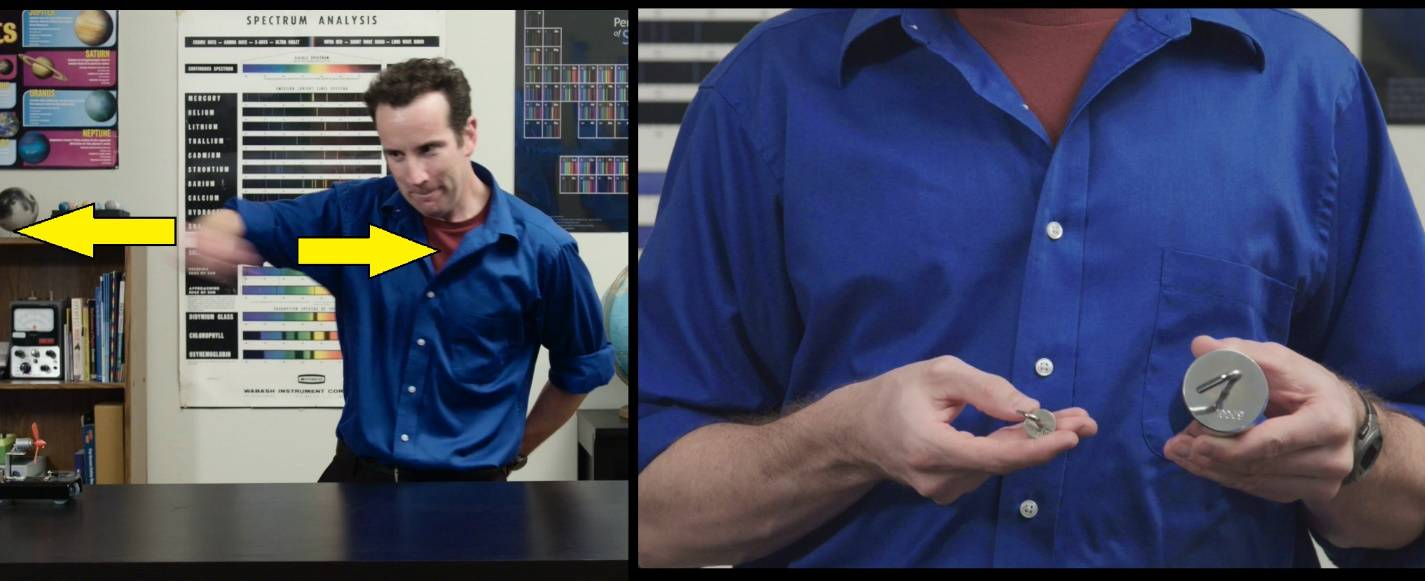Figure 4. Shaking objects quickly can demonstrate inertia, which is measured by mass. I show that a 10 g mass can be rapidly shaken, but not a 1 kg mass.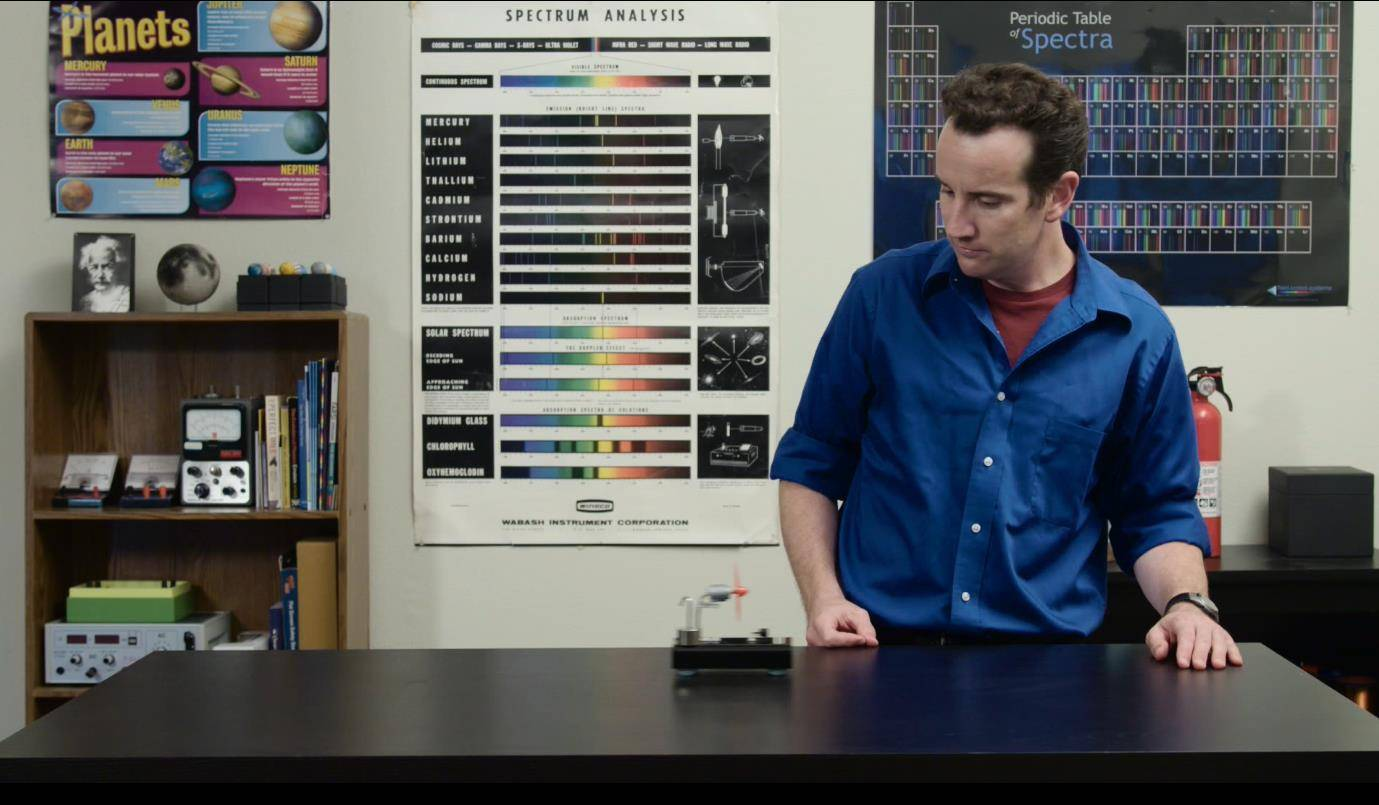Figure 5. A Fan Cart can be loaded with mass to demonstrate that the same force causes less acceleration. This is an excellent demonstration but is only half of the story of F=ma.

Figure 6. The Inertial Balance set can be used to demonstrate that even inanimate objects, like these metal strips, can have a difficult time bouncing larger amounts of mass around.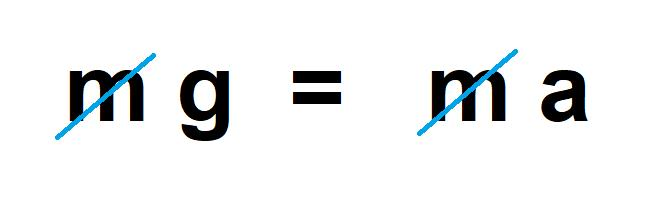A massive object m has a greater weight, due to the force of gravity mg. But that same object also has more inertia, also symbolized by m, and that makes it harder to move around, even for the earth’s gravity. These two cancel out and the acceleration, is simply g.

Now you might not believe that an inanimate object like the earth could have trouble moving things around, but that is precisely the case. Here, I have an Inertial Balance, which shows you that more massive objects are harder to move about even for objects like tables and springs or metal bands. The rate at which it can bound the greater inertia is less.

3rd Law

It is now time to state Newton’s 3rd law properly.

For every action force, there is a reaction force that is equal in magnitude but opposite in direction.

In Newton’s words, when you push on a stone, the stone pushes equally back on your finger.

It is possible to show the third law more accurately. Set up a situation where two people pull on the same spring scale, their scales will always agree.

What if you ask your partner to pull with 20N and you only pull with 10 N. This is impossible to achieve, but the result will be that they will move in the direction of the person pulling harder.

Newton did discuss the tides and the motions of the planets, especially Jupiter, how it would perturb the sun, causing it to wobble. But he did not know about Pluto, which is a great way to illustrate the third law in terms of gravitation.

There is also Newton’s own example, the cart and the horse. In the Principia, Isaac Newton discussed how his 3rd Law can best be understood in the context of a horse pulling on a cart. Paul Hewitt immortalized this example still further by using it as the basis of a cartoon in his masterpiece book called Conceptual Physics.

Newton’s third law explains jumping. When I jump I push down on the ground with my feet, which pushes me upward with a force equal to my push.

A lot of the names we give for exercises seem to violate Newton’s 3rd Law. For example, have you ever done a pull-up, what are you doing to the bar when you do this? Pulling down! It is the bar that pulls you up! Have you ever done a push-up? What are you doing when you do this? Yes, pushing down, the ground is doing the push-up.
Another way to understand Newton’s third law is through the example of the Air-Powered Projectile. When a burst of air is pushed out of the bottom of the tube, the projectile is pushed up by that same air. All rockets work on this principle, but the air-powered projectile is not truly a rocket because it only ejects the gas once and provides no flame, etc.

Newton’s 4th Law

Now, what about the planets, Jupiter, and the tides? Newton explained that gravity reaches up from the surface of the earth and out into space, expanding and diminishing.

The acceleration of gravity weakens in proportion to the distance squared.

The gravitational field of a massive planet extends through space, but all the while weakens as the square of the distance from that planet. The distance is initially measured to be the radius of the planet, so the gravity would not be twice as weak when you are an extra meter above the surface, but a full earth’s radius.

This is usually written similar to the form of F=GMm/r2 and most people incorrectly believe that Newton didn’t know the value G, but that is another Isaac Newton myth.

Newton guessed that the density of the earth was between 5 and 6 times that of water. Which is correct. He then used the volume of a sphere to estimate the mass from mass = Density times "bulk", Newton's word for volume. Most people who teach physics are aware of Henry Cavendish’s experiment to determine the universal constant G. Except, that he was interested in measuring the density of the earth. The symbol G was introduced much later.

The one thing that Newton could not understand was how gravity acted through space. We now better understand that it is a gravity field that extends from the sun to the planets. This is the Einsteinian model, that mass bends the fabric of space-time, recently confirmed by the detection of gravitational waves that distort the gravitational field and travel at the speed of light.

One of the best ways to demonstrate the modern answer to Newton’s conundrum about “occult forces” and through the Einsteinian Model of the warping of space, time is with the Gravity Well. This device is surprisingly easy to build. I have my own set of planets which I like to send around the large 1 kg sun ball that is provided. However, the set comes with colored marbles which will illustrate the point just as well.

The corrections made by Einstein to Newton’s gravity theory were very slight, but they were non-negligible. There were three specific predictions.

1. Light would be bent by gravity a very specific amount.
2. The orbit of Mercury would process (spin) over many years.
3. There would be gravity waves that would travel at the speed of light.

The first of these was demonstrated during a solar eclipse, two years after the publication of Einstein’s Theory of General Relativity. The Newton theory predicted less deviation of light than the Einstein theory. The experiment was done by Arthur Eddington, taking pictures of stars during a solar eclipse. Their positions were deflected by the sun’s gravity.

The second of these errors was already known to astronomers. Because the sun is not the only object in the solar system, the other planets deflect one another from their orbital paths. The orbit of Mercury is an ellipse, but this ellipse precesses slightly over the centuries (Figure 16). Newton's theory also predicts this (due to the presence of other planets) but Einstein's theory worked better. The error was about 1 degree over 2 years. This error is called "precession of the perihelion." Einstein correctly reasoned that Mercury would show the biggest error when compared to the Newton solution because it was so close to the sun. He was right, and so was his new theory.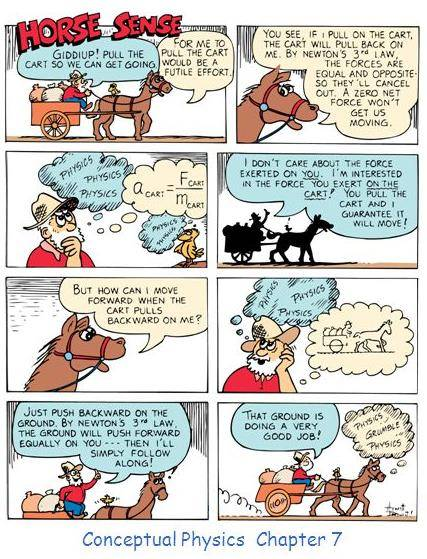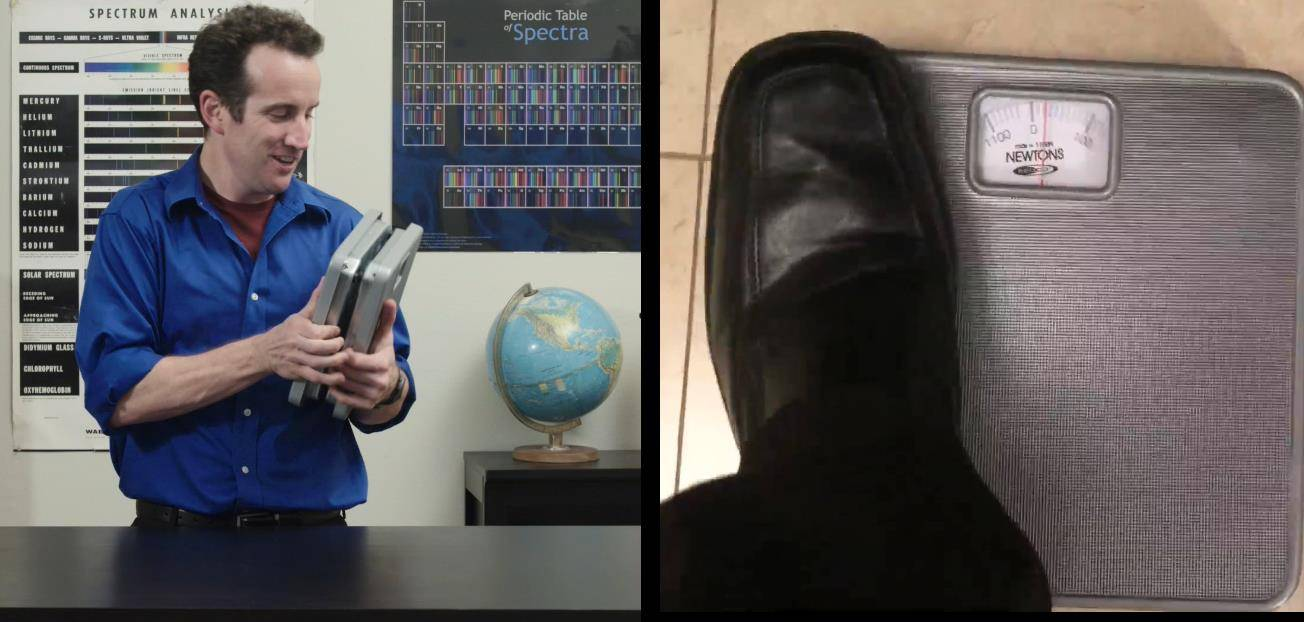Figure 8. It is possible to demonstrate Newton’s 3rd Law by putting two Newton scales together. These are quite funny, they told me that I weight 800N.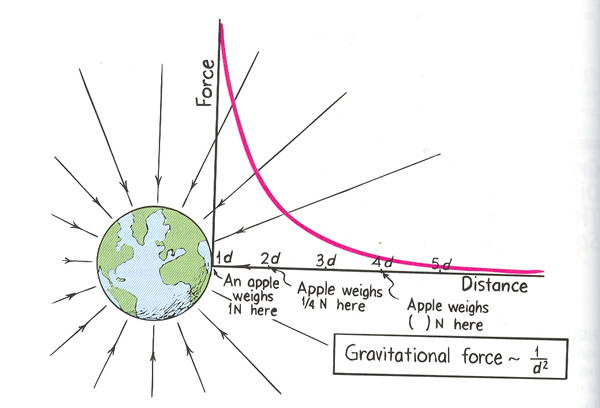Figure 9. The gravitational field of a planet extends through space and can be modeled by an equation.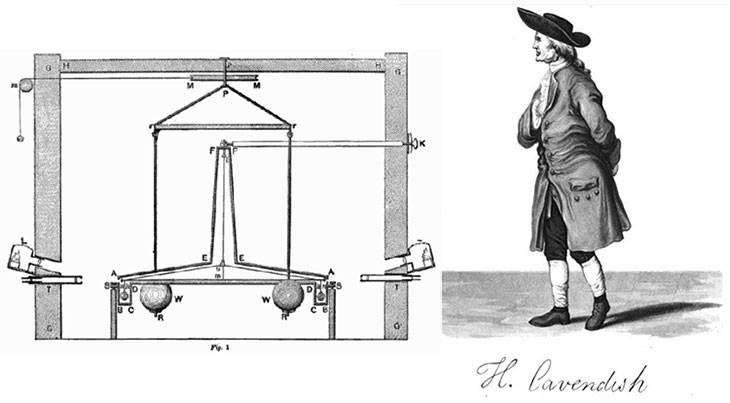Figure 10. This device is called a torsion balance. Two heavy weights, W, were attracted by the two little weights, C. A telescope, T, was used to observe the twisting of the rope. Fun fact, Henry Cavendish is also the discoverer of Hydrogen.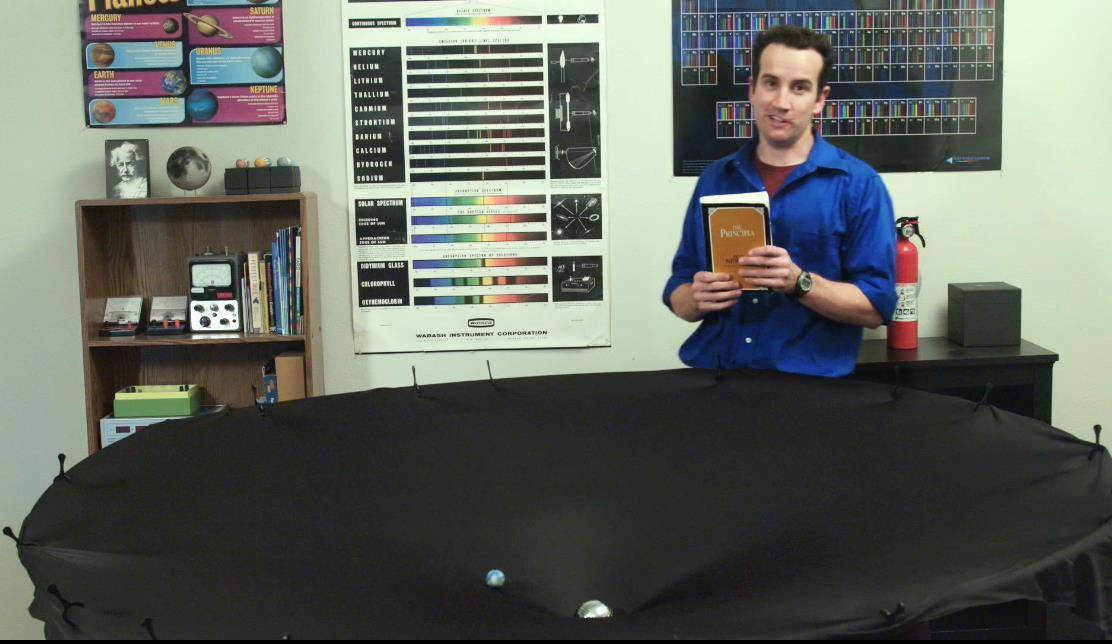Figure 11. The warping of space-time is the current best explanation for how gravitational forces seem to reach out and pull on masses. In Newton’s time, he had to appeal to the idea that since the law worked, it must be true.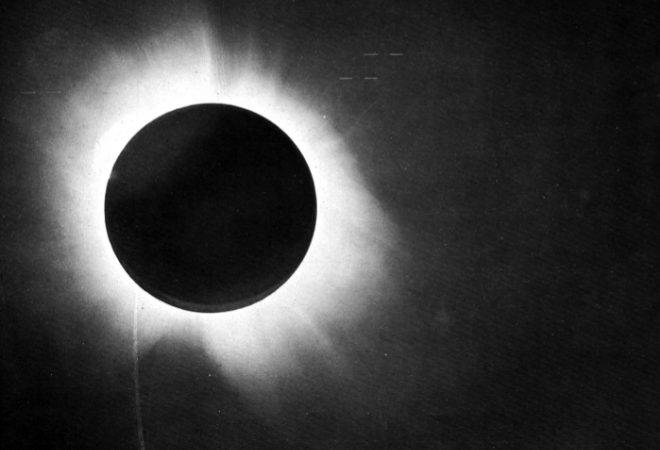Figure 12. Arthur Eddington’s famous photograph of stars during a solar eclipse proved that Einstein’s new theory was more accurate than Newton’s old one.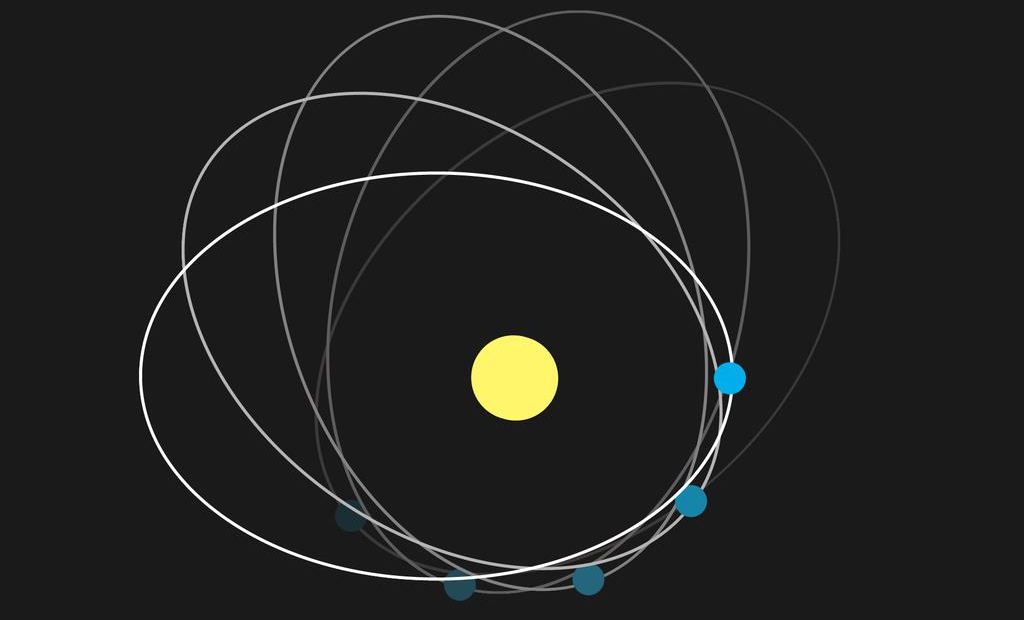Figure 16. Precession of the perihelion.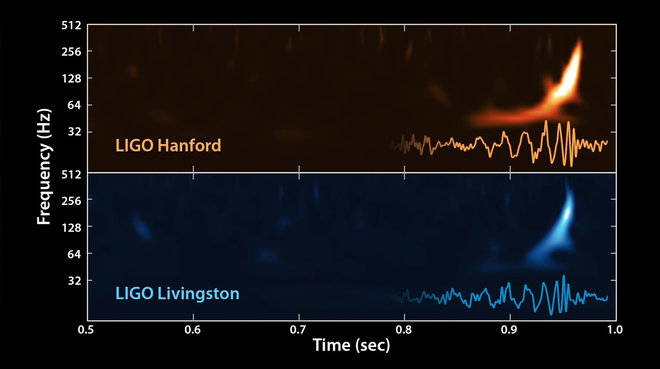Figure 17. Shown is the graph of the two detectors in Hanford and Livingston, respectively. Note, the frequencies measured are inaudible.

The third prediction of general relativity is that there would be gravitational waves that would propagate at the speed of light. This was demonstrated only as recently as 2016 in the LIGO laboratories and has already been rewarded with a Nobel Prize. These gravitational waves were detected twice, and the signal was the same. The event was supposedly a pair of black holes colliding in another galaxy and sending ripples through the fabric of space-time (Figure 17).

These such vibrations can also be demonstrated with the Gravity Well. Here is a video in which they are demonstrated with a drill. A strobe light is used to help make the waves more visible.

James Lincoln